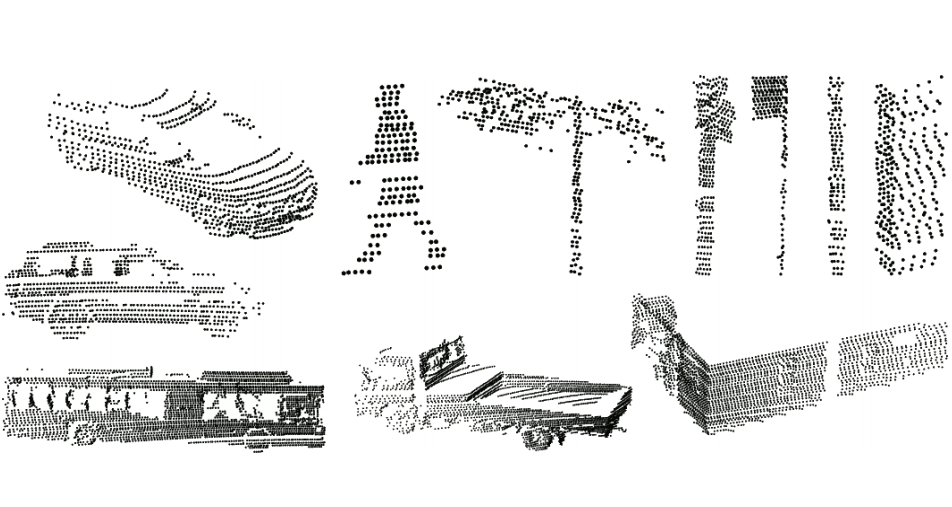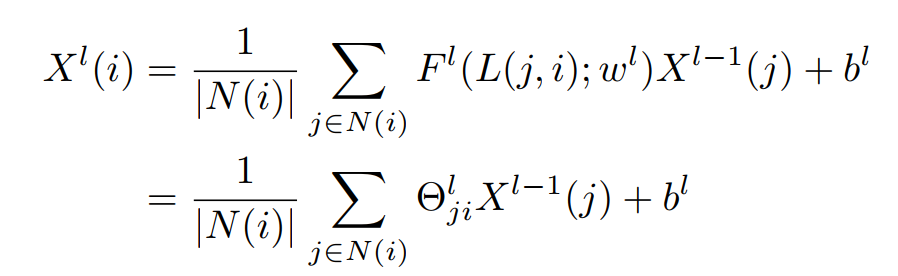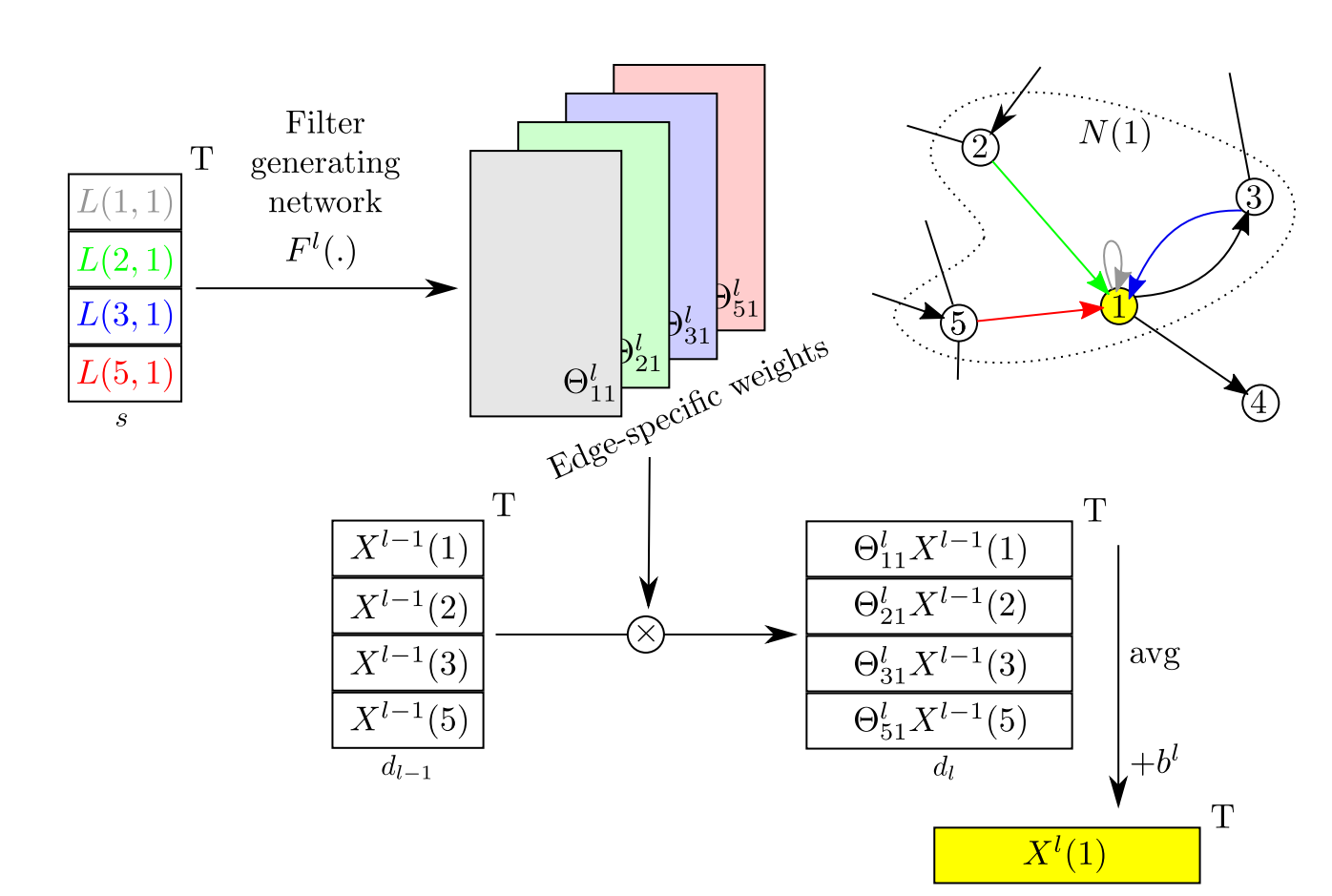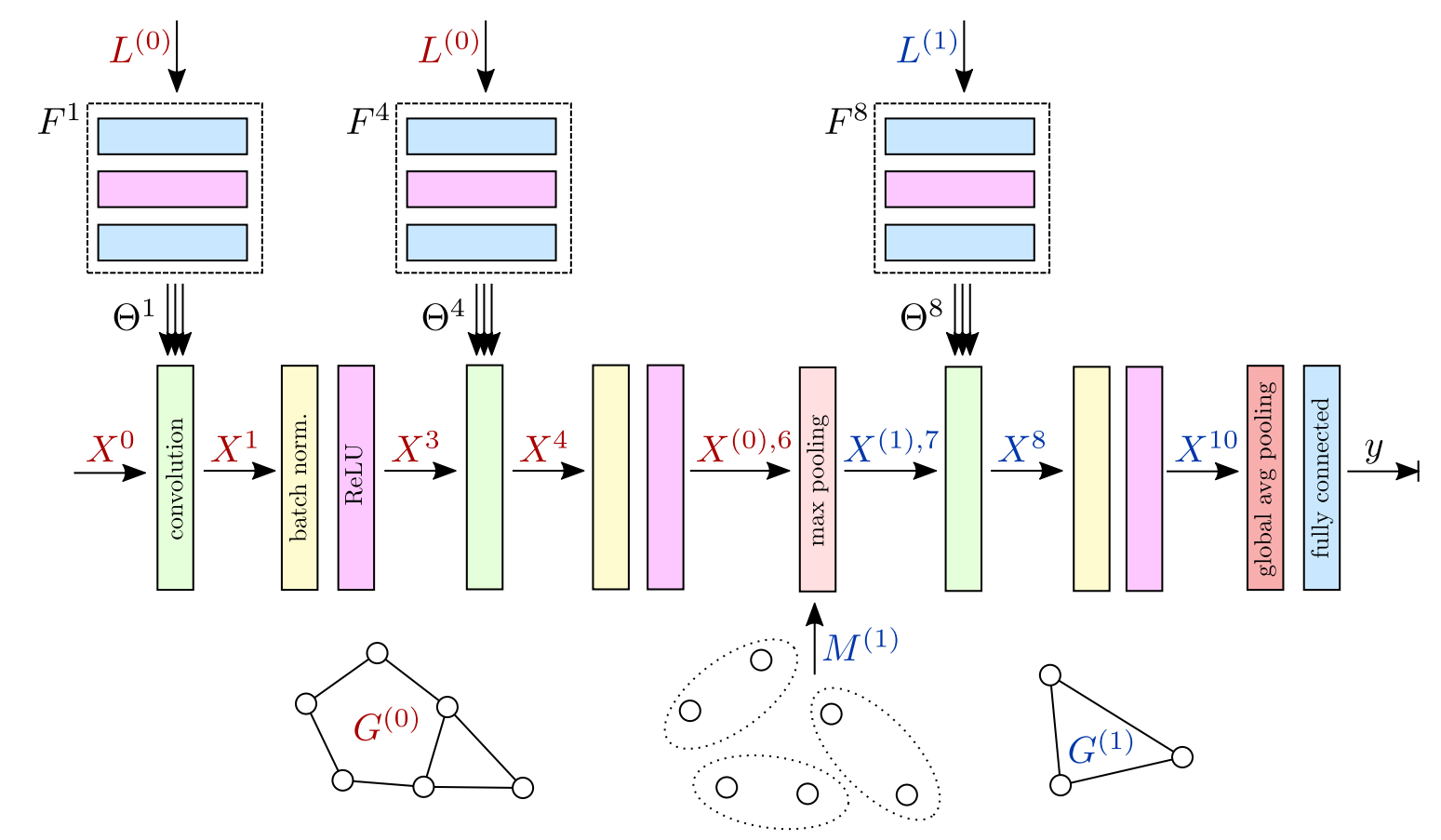In this paper, the authors perform point cloud classification (like image classification) on graph whose configuration is not fixed. A graph whose configuration is not fixed is one for which the vertices do not have the same number of edges. The graph is constructed from a point cloud (typically obtained with a lidar scanner) and a pre-processing remeshing operation.Since the number of edges is not the same for each vertices (unlike an image for example) they propose a new type of convolution operator which involves a function $$F$$ which converts an edge label $$L$$ and the weight vector $$w$$ to a floating point value $$\Theta$$. Note that in Fig.2, the use of $$F(L,w)$$ allows to obtain a normal convolution (second equation). Note that $$X$$ are feature maps, $$l$$ is the layer index, $$w^l$$ is the weight vector of layer $$l$$, and $$b^l$$ is the bias of layer $$l$$.The overall graph-CNN has the same structure than a conventional CNN except for the MaxPooling operation which involves a graph reduction algorithm ($$M^{(1)}$$ in the next figure).